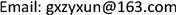﻿ 主体意图的结构模型及其实现模式研究 Research on the Structural Model of Subject Intention and Its Realization Model

Vol. 09  No. 06 ( 2019 ), Article ID: 31102 , 6 pages
10.12677/AG.2019.96063

Research on the Structural Model of Subject Intention and Its Realization Model

Yexun Zhong1,2,3,4, Baoqing Hu2,3,4, Zhongmei Li1, Jiaqi Liu1

1Department of Navigation Engineering, Naval University of Engineering, Wuhan Hubei

2Nanning Normal University, Nanning Guangxi

3Key Laboratory of Beibu Gulf Environment Change and Resources Use of Ministry of Education, Nanning Guangxi

4Guangxi Key Laboratory of Earth Surface Processes and Intelligent Simulation, Nanning GuangxiReceived: Jun. 7th, 2019; accepted: Jun. 21st, 2019; published: Jun. 28th, 2019ABSTRACT

Planning, design and scheme of related projects are the main intention to achieve certain goals. These plans, designs and schemes have common structural characteristics and basic elements: expected quality objectives, planned time limit, planned capital, planned material consumption and expected economic benefits. The process from conceptual model to physical engineering is a topological mapping. The basic elements are transformed into actual elements by topological mapping: actual quality, actual construction period, actual use of funds, actual material consumption and actual economic benefits. According to the difference between actual elements and planned elements, the factors for evaluating related projects can be deduced: the high or low quality of the project, the long or short duration of the project, the use of funds, material consumption, economic benefits and other factors. By using the set of evaluation factors, we can make a comprehensive evaluation of related projects. The mathematical definition of innovation concept and its scientific value are put forward. This paper expounds the necessity and importance of integrating innovative thinking in relevant planning projects.

Keywords:Concept Model, Planning, Topological Mapping, Basic Elements, Innovation, Evaluation Factor

1海军工程大学导航工程系，湖北 武汉

2南宁师范大学，广西 南宁

3北部湾环境演变与资源利用省部共建教育部重点实验室，广西 南宁

4广西地表过程与智能模拟重点实验室，广西 南宁1. 引言

2. 从拓扑映射观点看观念模型的物化

【定义1】映射  设f是从X到Y的关系，即 $f\subset X×Y$ 。如果 $\forall x\in X$ ，存在唯一的 $y\in Y$ 使 $\left(x,y\right)\in f$ ，则称f是从X到Y的映射(函数)，记作 $f:X\to Y$ ，而称X是f的定义域，若 $\left(x,y\right)\in f$ ，则用 $y=f\left(x\right)$ 表示，并称y是在f下的像或值。

3. 规划工程及其实践的基本构成元素

3.1. 规划工程的基本构成元素

1) 期望质量目标：质量目标是项目完成后要达到的质量要求：

${Z}_{I}^{a}\in {G}^{a}\in X$ (1)

2) 计划完成时间(工期)：设计划的完成时间为：

${T}_{I}^{a}\in {G}^{a}\in X$ (2)

3) 计划投入资金：设计划投入资金为：

${J}_{I}^{a}\in {G}^{a}\in X$ (3)

4) 计划材料消耗：设计划材料消耗为：

${O}_{I}^{a}\in {G}^{a}\in X$ (4)

5) 计划经济效益：设计划经济效益为：

${K}_{I}^{a}\in {G}^{a}\in X$ (5)

3.2. 实际工程的基本构成元素

${G}^{b}=f\left({G}^{a}\right)|{G}^{a}\in X,{G}^{b}\in Y$ (6)

1) 实际质量：实际质量是质量目标 ${Z}_{I}^{a}$ 在f下的映像：

$f\left({Z}_{I}^{a}\right)={Z}_{I}^{b}$ (7)

2) 实际完成时间(工期)：实际完成时间是计划的完成时间 ${T}_{I}^{a}$ 在f下的映像：

$f\left({T}_{I}^{a}\right)={T}_{I}^{b}$ (8)

3) 实际投入资金：实际投入资金是计划投入资金 ${J}_{I}^{a}$ 在f下的映像：

$f\left({J}_{I}^{a}\right)={J}_{I}^{b}$ (9)

4) 实际材料消耗：实际是材料消耗是计划材料消耗 ${O}_{I}^{a}$ 在f下的映像：

$f\left({O}_{I}^{a}\right)={O}_{I}^{b}$ (10)

5) 实际经济效益：实际经济效益是计划经济效益 ${K}_{I}^{a}$ 在f下的映像：

$f\left({K}_{I}^{a}\right)={K}_{I}^{b}$ (11)

3.3. 基于实际指标与期望指标的贴近度派生的评价因子

【定义2】高质量工程与不合格工程 $\exists {Z}_{I}^{a}\in {G}_{I}^{a}$${Z}_{I}^{b}\in {G}_{I}^{b}$ ，若 ${Z}_{I}^{b}$${Z}_{I}^{a}$ 之差满足：

${Z}_{I}^{b}-{Z}_{I}^{a}=0⇔合格工程$ (12)

${Z}_{I}^{b}-{Z}_{I}^{a}<0⇔优质工程$ (13)

${Z}_{I}^{b}-{Z}_{I}^{a}>0⇔不合格工程$ (14)

【定义3】完成工程的时间效率 $\exists {T}_{I}^{a}\in {G}_{I}^{a}$${T}_{I}^{b}\in {G}_{I}^{b}$ ，若 ${T}_{I}^{b}$${T}_{I}^{a}$ 之差满足：

${T}_{I}^{b}-{T}_{I}^{a}=0⇔工程按时完成$ (15)

${T}_{I}^{b}-{T}_{I}^{a}<0⇔工程提前完成$ (16)

${T}_{I}^{b}-{T}_{I}^{a}>0⇔工程延期完成$ (17)

【定义4】资金使用状况 $\exists {J}_{I}^{a}\in {G}_{I}^{a}$${J}_{I}^{b}\in {G}_{I}^{b}$ ，若 ${J}_{I}^{b}$${J}_{I}^{a}$ 之差满足：

${J}_{I}^{b}-{J}_{I}^{a}=0⇔预算与实际一致$ (18)

${J}_{I}^{b}-{J}_{I}^{a}<0⇔节约了资金$ (19)

${J}_{I}^{b}-{J}_{I}^{a}>0⇔超预算使用$ (20)

【定义5】材料消耗状况 $\exists {O}_{I}^{a}\in {G}_{I}^{a}$${O}_{I}^{b}\in {G}_{I}^{b}$ ，若 ${O}_{I}^{b}$${O}_{I}^{a}$ 之差满足：

${O}_{I}^{b}-{O}_{I}^{a}=0⇔预算与实际一致$ (21)

${O}_{I}^{b}-{O}_{I}^{a}<0⇔节约了材料$ (22)

${O}_{I}^{b}-{O}_{I}^{a}>0⇔超预算使用材料$ (23)

【定义6】经济效益 $\exists {K}_{I}^{a}\in {G}_{I}^{a}$${K}_{I}^{b}\in {G}_{I}^{b}$ ，若 ${K}_{I}^{b}$${K}_{I}^{a}$ 之差满足：

${K}_{I}^{b}-{K}_{I}^{a}=0⇔预算与实际一致$ (24)

${K}_{I}^{b}-{K}_{I}^{a}<0⇔经济效益差$ (25)

${K}_{I}^{b}-{K}_{I}^{a}>0⇔经济效益好$ (26)

4. 创新概念及其科学价值

4.1. 创新的数学定义

【定义7】创新：设 ${t}_{0j}\in T$${S}_{J}={\cup }_{j\in J}{S}_{j}$ ，若下列条件满足：

$\forall t<{t}_{0}⇒\nexists {S}_{j},t={t}_{0}⇒{S}_{j}\ne \varnothing \wedge \forall t>{t}_{0}⇒\exists {S}_{j}$ (27)

4.2. 创新的科学价值

5. 结语

Research on the Structural Model of Subject Intention and Its Realization Model[J]. 地球科学前沿, 2019, 09(06): 590-595. https://doi.org/10.12677/AG.2019.96063

1. 1. 汪信砚. 科学价值论[M]. 桂林: 广西师范大学出版社, 1995.

2. 2. 李述一. 理想世界的创造[M]. 北京: 人民出版社, 1988.

3. 3. 欧文•拉兹洛. 系统, 结构和经验[M]. 上海: 上海译文出版社, 1987.

4. 4. 程吉树, 陈水利. 点集拓扑学[M]. 北京: 科学出版社, 2008.

5. 5. 钟业勋, 胡宝清, 边少锋, 等. 地图审校和错误修改的数学定义及其应用[J]. 海洋测绘, 2015, 35(2): 26-28.

6. 6. 钟业勋, 胡宝清, 边少锋, 等. 地图审校和错误修改的数学定义及地图质量控制模型[J]. 桂林理工大学学报, 2015, 35(2): 343-347.

7. 7. 钟业勋, 胡宝清. 数理地图学(第二版) [M]. 北京: 测绘出版社, 2017.

8. 8. 钟业勋, 胡宝清. 物质存在的时空条件和事物演化的数学模型[J]. 玉林师范学院学报, 2013, 34(5): 144-148.

9. 9. 毕思文. 地球系统科学(第二版) [M]. 北京: 科学出版社, 2003.

10. 10. 丹尼尔•卡拉曼尼. 基于布尔代数的比较法导论[M]. 蒋勤, 译. 上海: 格致出版社, 上海人民出版社, 2012.

11. 11. 韦清嫄, 钟业勋, 胡宝清, 等. 地物物理量的度量模型及其用[J]. 桂林理工大学学报, 2015, 35(1): 117-120.

12. 12. 钟业勋, 胡宝清, 童新华, 等. 地图学概念的数学表述研究[M]. 北京: 科学出版社, 2015.

13. 13. 钟业勋. 我的科研情怀和人生感悟[C]//海军工程大学. 国家自然科学基金项目评审暨地图投影学术交流会, 2015.

14. 14. 钟业勋. 吻接圆弧边经线多圆锥投影: 预先给定变形分布设计多圆锥投影方法的探讨[C]//吴忠性, 胡毓钜. 地图投影论文集. 北京: 测绘出版社, 1983: 230-254.

15. 15. 边少锋, 李厚朴, 李忠美. 地图投影计算机代数分析研究进展[J]. 测绘学报, 2017, 46(10): 1557-1569.